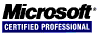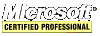## Radix Sort Algorithm in C/C++

Radix sort appears to sort numbers very well indeed; however, it does not seem applicable to strings. Plus, quick sort out performs it. See the Algorithm Study for comparisons.

``````// more or less, the original algorithm as posted on Wikipedia
{
private:
static void rad_sort_u(unsigned int *from, unsigned int *to, unsigned int bit)
{
if (!bit || to < from + 1) return;

unsigned int *ll = from, *rr = to - 1, tmp;
while (1) {
while (ll < rr && !(*ll & bit)) ll++;
while (ll < rr &&	(*rr & bit)) rr--;
if (ll >= rr) break;
tmp = *ll;
*ll = *rr;
*rr = tmp;
}

if (!(bit & *ll) && ll < to) ll++;
bit >>= 1;

}
public:
static void radix_sort(int *array, const unsigned int len)
{
unsigned int i, *x = (unsigned int*)array;

for(i = 0; i < len; i++)
x[i] ^= -2147483648;